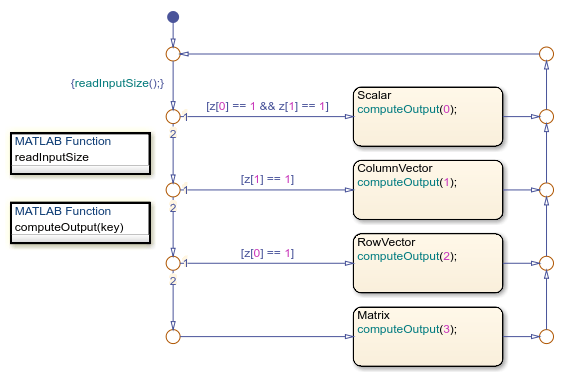## Declare Variable-Size Data in Stateflow Charts

The size of most data in a Stateflow® chart in a Simulink® model is fixed at compile time and does not change at run time. In contrast, variable-size data can change size during simulation. For example, you can use variable-size data if the output from a Stateflow chart is an array whose size depends on the state of the chart.

### Enable Support for Variable-Size Data

By default, Stateflow charts support variable-size data. To enable or disable this support for individual charts, modify the chart property Support variable-size arrays, as described in Specify Properties for Stateflow Charts. For more information, see Support variable-size arrays.

When you enable this chart property, your chart accepts variable-size input data from other blocks in the Simulink model. You can declare additional variable-size data objects by enabling the Variable size data property, as described in Set Data Properties. For more information, see Variable size.

Use the Size data property to explicitly specify the maximum size for each variable-size data object. For example, to specify a 2-D matrix where the maximum size is 2 for the first dimension and 4 for the second dimension, enter `[2 4]`. To set an array as unbounded, set the Size data property to `inf`. For example, for a 2-D matrix whose size does not have a maximum, set `[inf,inf]`.

Alternatively, to inherit the maximum size of data, set Size to `-1`. For more information, see Specify Size of Stateflow Data.

Note

You cannot enable variable-size data for scalar data.

### Variable-Size Data in Charts That Use MATLAB as the Action Language

Charts that use MATLAB® as the action language support variable-size chart-level input, local, and output data, as well as variable-size input and output data in graphical functions, MATLAB functions, and truth table functions.

You can modify variable-size chart data in state and transition actions. For example, in this chart that uses MATLAB as the action language, the state actions assign the variable-size chart output, `y`, to values that range in size from a scalar to a 2-by-4 matrix.### Variable-Size Data in Charts That Use C as the Action Language

Charts that use C as the action language support variable-size chart-level input and output data, as well as variable-size input, local, temporary, and output data in MATLAB functions and truth table functions that use MATLAB as the action language.

You can only modify variable-size chart data by using:

• MATLAB functions

For example, in this chart that uses C as the action language, the MATLAB function `readInputSize` determines the size of the variable-size chart input. Then, the MATLAB function `computeOutput` applies a size-dependent algorithm to the chart input and sets the value of the variable-size chart output.